# Keeping the distance or spans of continua Alex

• Slides: 36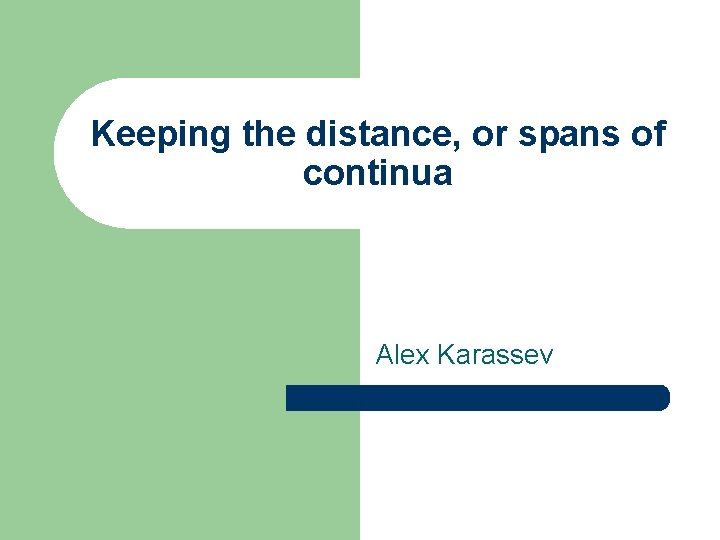Keeping the distance, or spans of continua Alex KarassevWhat is a continuum? l In the plane or space: an object which is bounded q closed q connected qBounded diameter Bounded UnboundedClosed Non-closed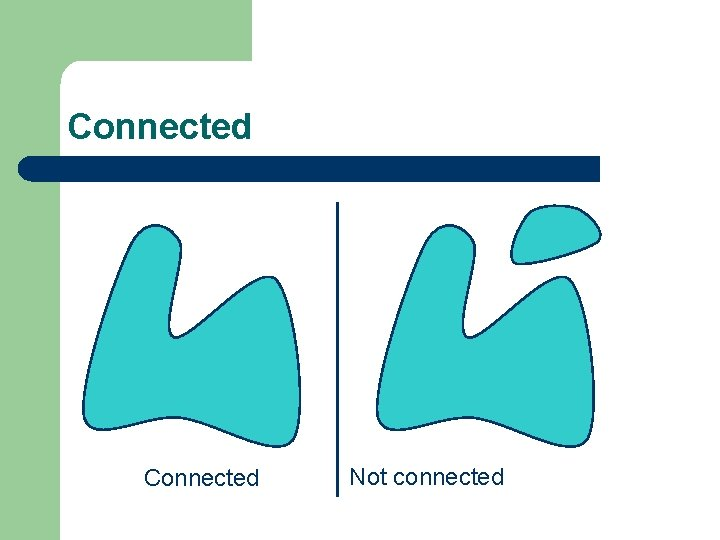Connected Not connected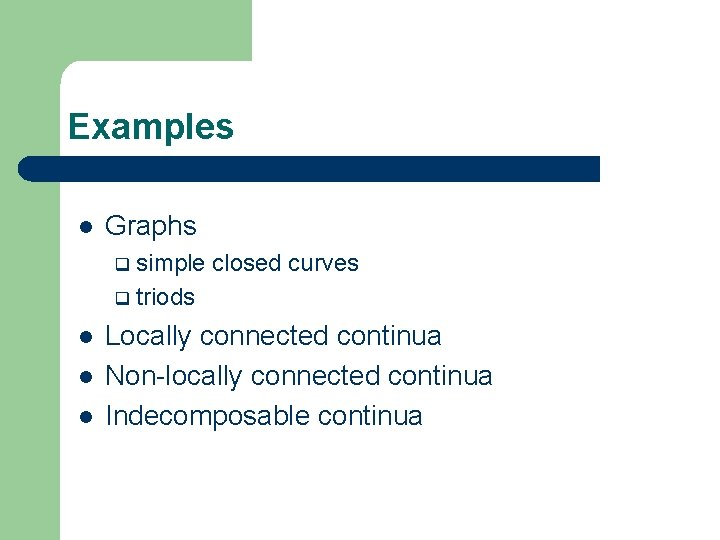Examples l Graphs simple closed curves q triods q l l l Locally connected continua Non-locally connected continua Indecomposable continuaSimple closed curvesTriods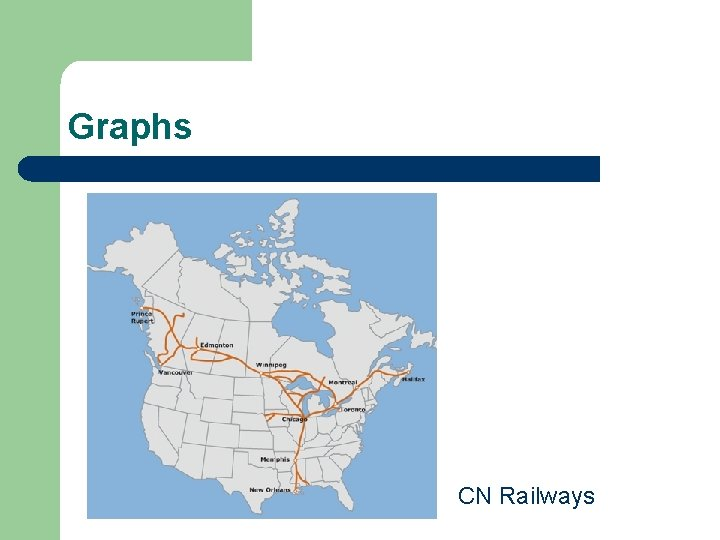Graphs CN Railways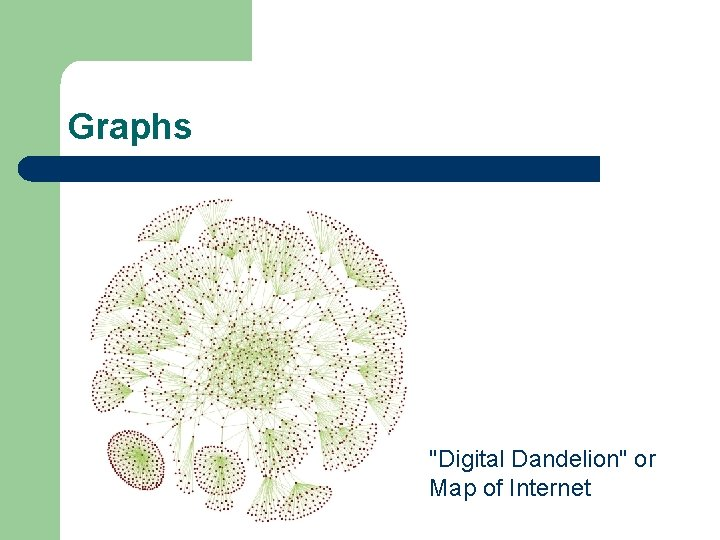Graphs "Digital Dandelion" or Map of Internet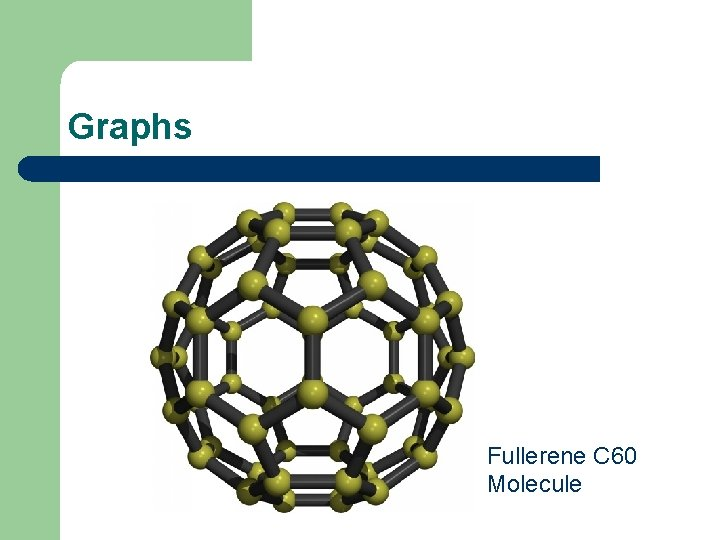Graphs Fullerene C 60 Molecule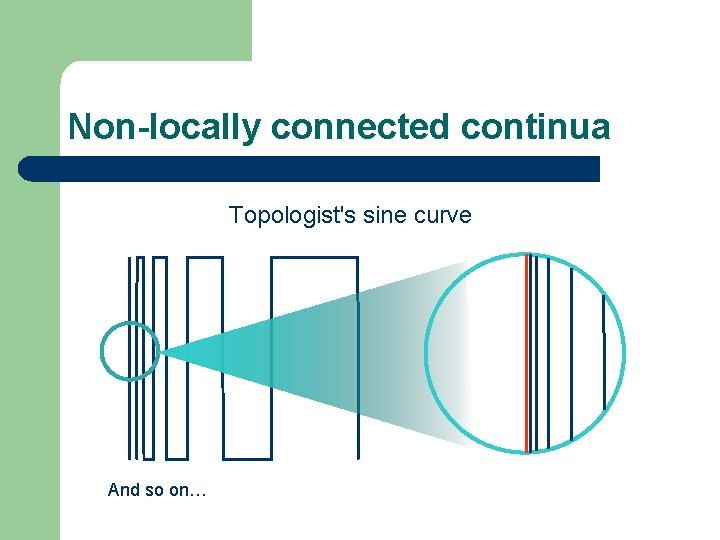Non-locally connected continua Topologist's sine curve And so on…Decomposable continua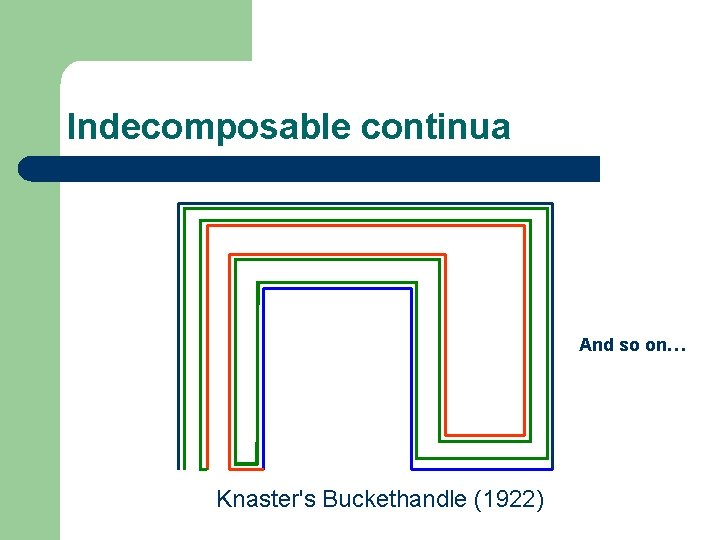Indecomposable continua And so on… Knaster's Buckethandle (1922)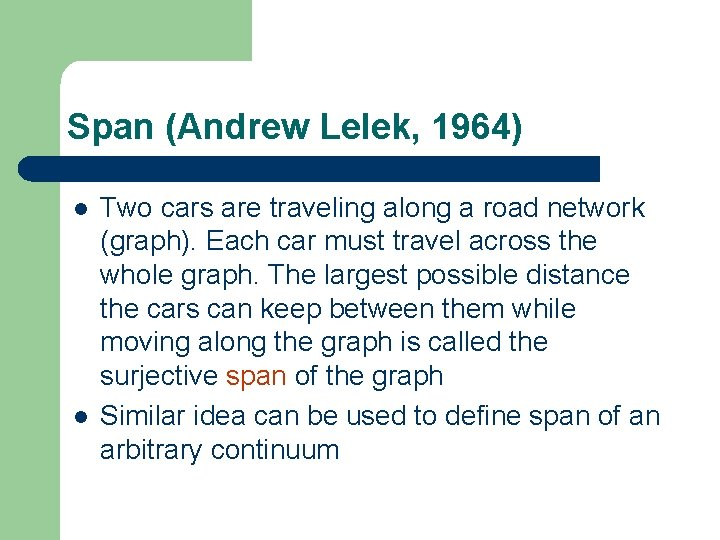Span (Andrew Lelek, 1964) l l Two cars are traveling along a road network (graph). Each car must travel across the whole graph. The largest possible distance the cars can keep between them while moving along the graph is called the surjective span of the graph Similar idea can be used to define span of an arbitrary continuumCircle Span = diameterTriod l l l We can start at end points However, at some moment one of cars must reach the center So the largest possible value for span is the length of the leg span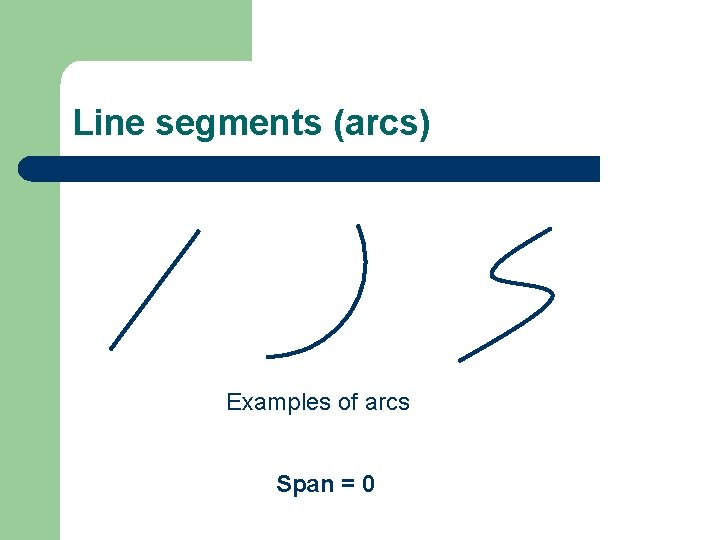Line segments (arcs) Examples of arcs Span = 0More complicated cases? Simple closed curve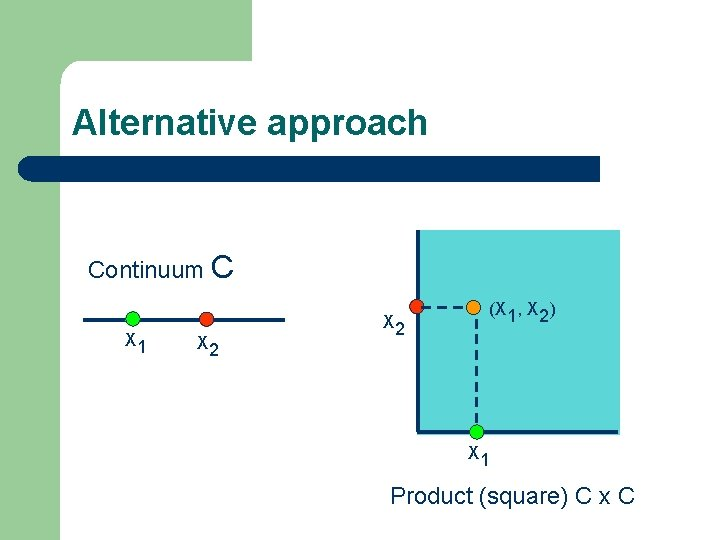Alternative approach Continuum C X 1 X 2 (X 1, X 2) X 1 Product (square) C x CAlternative approach Two paths in the continuum = one path in the square! (0, 1) 0 1 X 2 (1, 0)Representation of distances ce = 0) Distance < 1/2 an t s i x n o g ia ( al x = 1 , 2 d Distance = 1/2 D 0 1 In general, the set of points with distance < d is represented by a symmetric neighbourhood of the diagonal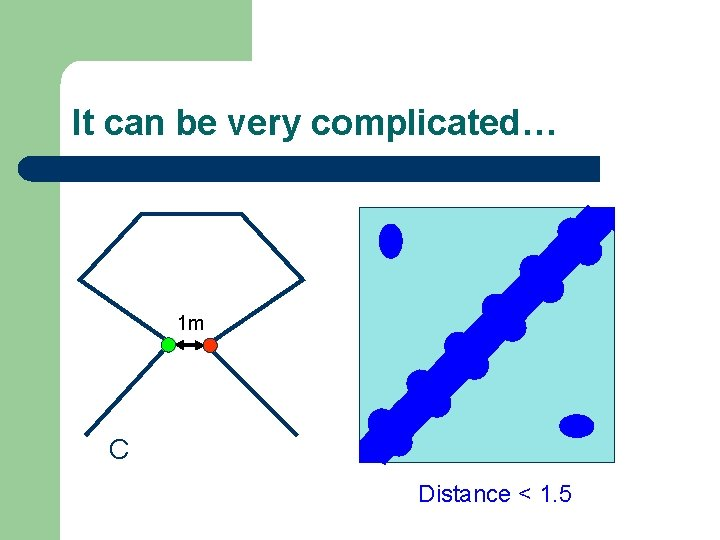It can be very complicated… 1 m C Distance < 1. 5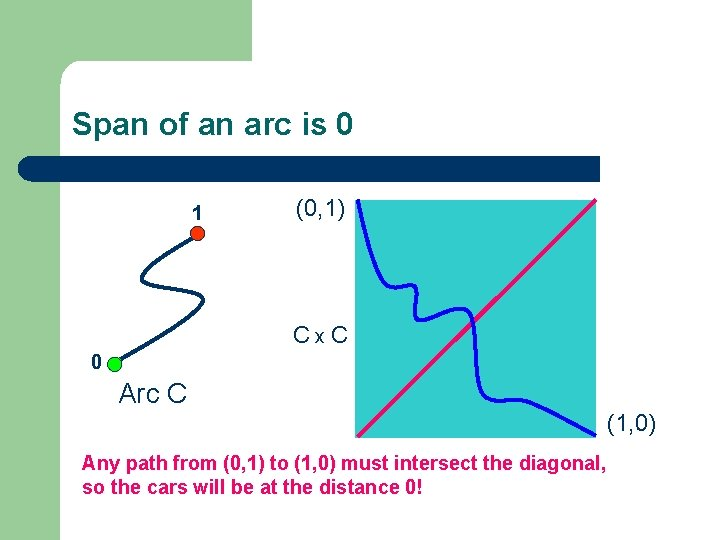Span of an arc is 0 1 (0, 1) Cx. C 0 Arc C Any path from (0, 1) to (1, 0) must intersect the diagonal, so the cars will be at the distance 0! (1, 0)Square of a simple closed curve C C x C = Torus DiagonalVisualizing the product: simple closed curve Torus can be viewed as a square with identified opposite sides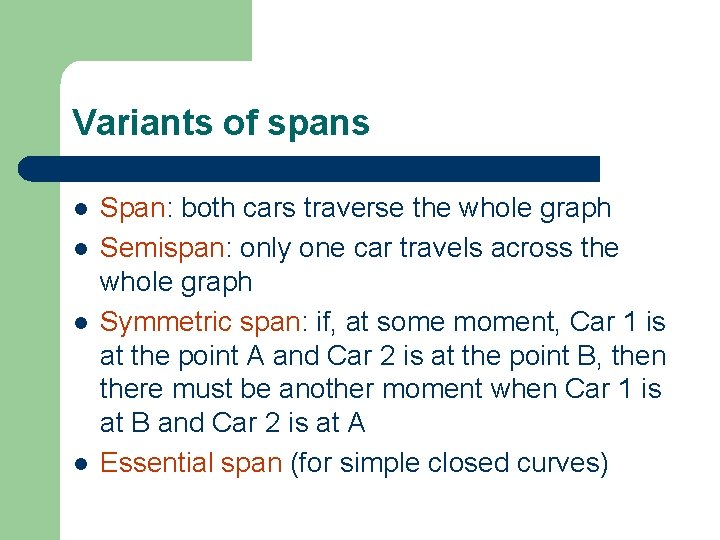Variants of spans l l Span: both cars traverse the whole graph Semispan: only one car travels across the whole graph Symmetric span: if, at some moment, Car 1 is at the point A and Car 2 is at the point B, then there must be another moment when Car 1 is at B and Car 2 is at A Essential span (for simple closed curves)Versions of span coincide for: circle "standard" triodQuestion of Andrew Lelek (1995) l Do different types of spans always agree for certain classes of continua, particularly for triods and simple closed curves?Some of our results Theorem (Logan Hoehn, Alex Karassev) No two of these versions of span agree for all simple closed curves or for all triods "Equivalent metrics and the spans of graphs", Colloquium Math, acceptedOur idea: predetermined distances l l Start with a neighbourhood of diagonal that have desired properties, and then define suitable metric This can be done by specifying closed sets in C x C which correspond to points with given distance (say 1 or 2)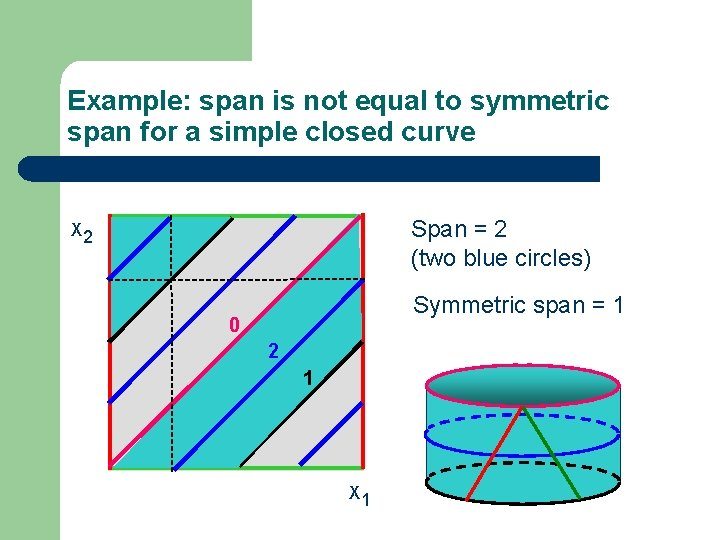Example: span is not equal to symmetric span for a simple closed curve Span = 2 (two blue circles) X 2 Symmetric span = 1 0 2 1 X 1Example: span is not equal to semispan for a simple closed curve Semispan = 2 (two blue arcs) X 2 <1 0 2 1 X 1 Span = 1Question of Howard Cook (1995) C 2 C 1 Is the span of C 1 less than the span of C 2?It seems very easy in the case of convex curves… d 1< d 2 C 2 d 1 C 1…but it is very complicated in general!